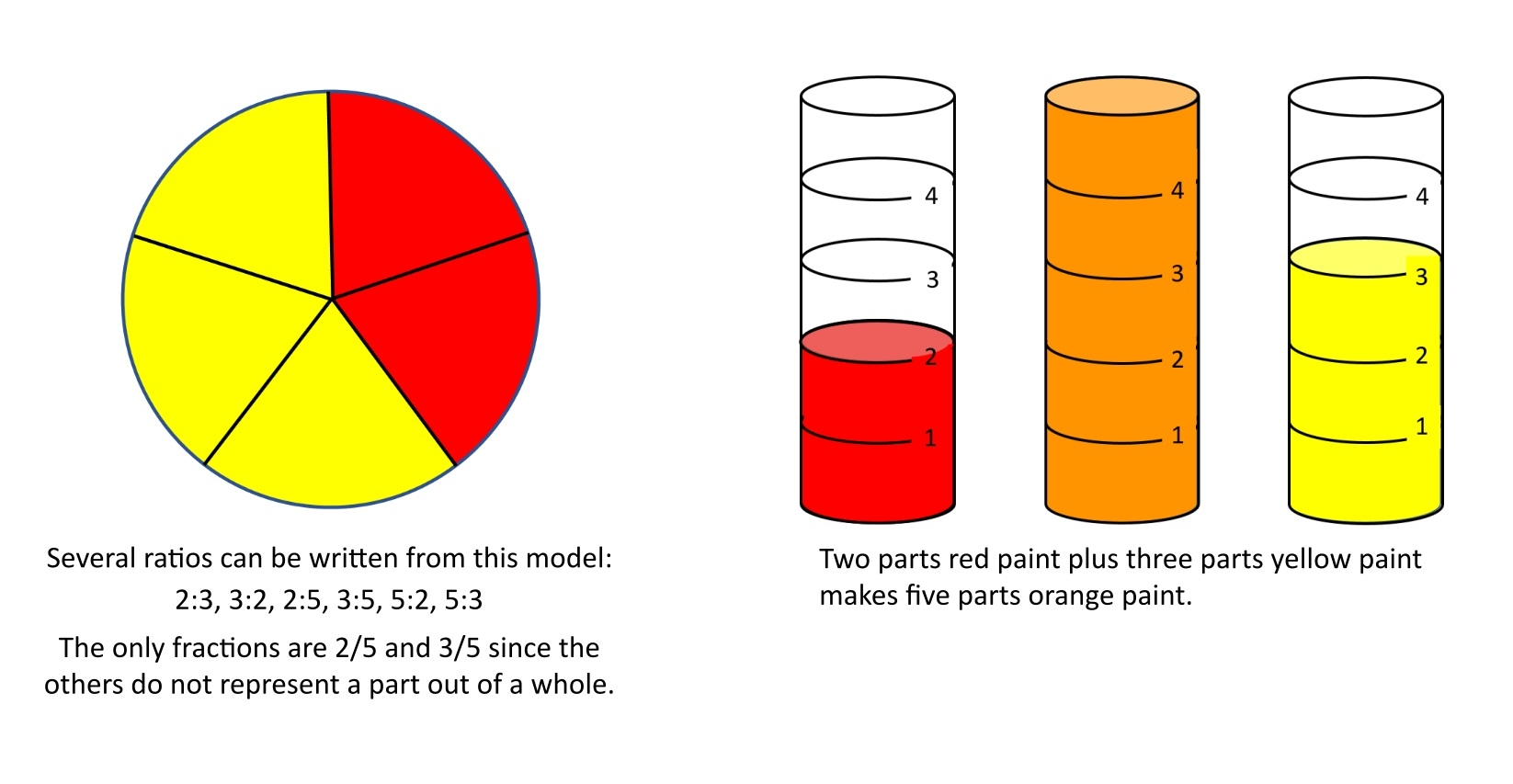By: David Bernasconi

When first introduced to fractions, students in the intermediate grades sometimes struggle to make sense of equivalence and fraction operations. By helping students develop a deep understanding of the role of factors in comparison and fraction operations, we also prepare them for topics that follow and that build upon this knowledge.

Many of the tools used to introduce these early fraction concepts can also be used later to help students recognize the relationships between fractions and ratio, rates, and proportions. Fractions with Prime Factor Tiles is the perfect tool to introduce and explore these concepts.

## Building Understanding of Ratios

A ratio is defined as the comparison of two numbers by division, a/b. Most ratios can be treated just like fractions when performing computations--whether the ratio is a part-to-whole or part-to-part comparison. Fractions and rates are simply special types of ratios. Fractions are exclusively part-to-whole comparisons, such as the portion of a pizza that was eaten, and rates represent comparisons between unlike quantities, such as the price of apples in dollars per pound. The distinctions will be much clearer for students if instruction is grounded in real-world problems and visual and physical models are employed. Without context or units, there is no way to tell ratios, rates, and fractions apart!## From Ratios to Rates

Including units of measure in computations and treating them as factors is a powerful way to perform conversions and model the relationship between ratios and rates. In the videos below, tiles bearing units of measure demonstrate how easy it is to make conversions and simplify ratios. Just as common numerical factors in a fraction multiplication problem are cancelled out, so too are unit factors. In simplifying a comparison of 9 feet to 2 yards, the units of length cancel out completely, resulting in a dimensionless ratio of 3/2 or 3:2.

## Simplifying a Ratio

In converting a rate of 25 yards-per-6 seconds into a rate of 25 feet-per- 2 seconds, the unit common to the numerator and denominator, yd, is cancelled out, and only the desired units, ft/s, remain.

## Proportional Relationships

Proportions are equivalent ratios, which makes setting up and solving them quite similar to generating equivalent fractions. Early exposure to proportions should be limited to problems with compatible numbers and real-world contexts to strengthen the connection to equivalent fractions, deepen understanding of the role of factors, and reinforce multiplication and division as inverse operations. In the next video, Prime Factor Tiles are used to generate equivalent fractions which leads naturally to solving a proportion for a missing value. Compatible numbers from the multiplication table make the process intuitive, and students apply either multiplication or division as the problem warrants.

## Solving Proportions for Missing Value

Proportions involving extended ratios, such as those found in recipe problems, can be confusing since they cannot be set up or solved like equivalent fractions. A visual model built from Prime Factor Tiles is easy to understand, intuitive to solve, and leads to a simple pencil and paper technique. Different measures are used for each ingredient in a recipe for fudge, but proportions can still be written using only the original ratio of 3:2:1.

## Conclusion

Fractions are indeed only the beginning! By using the same tool to introduce and reinforce related concepts, students are able to make connections and develop a deep understanding of fractions, ratios, and proportions.

For more applications of Prime Factor Tiles beyond fraction operations, check out the additional information including activities and videos at www.didax.com/pft.

# Limited Time Offer

Save 20% on Fractions with Prime Factor Tiles with promo code TILES20. Code expires 3/25/2019. Plus, don't forget, shipping is on us for all orders over \$99*.